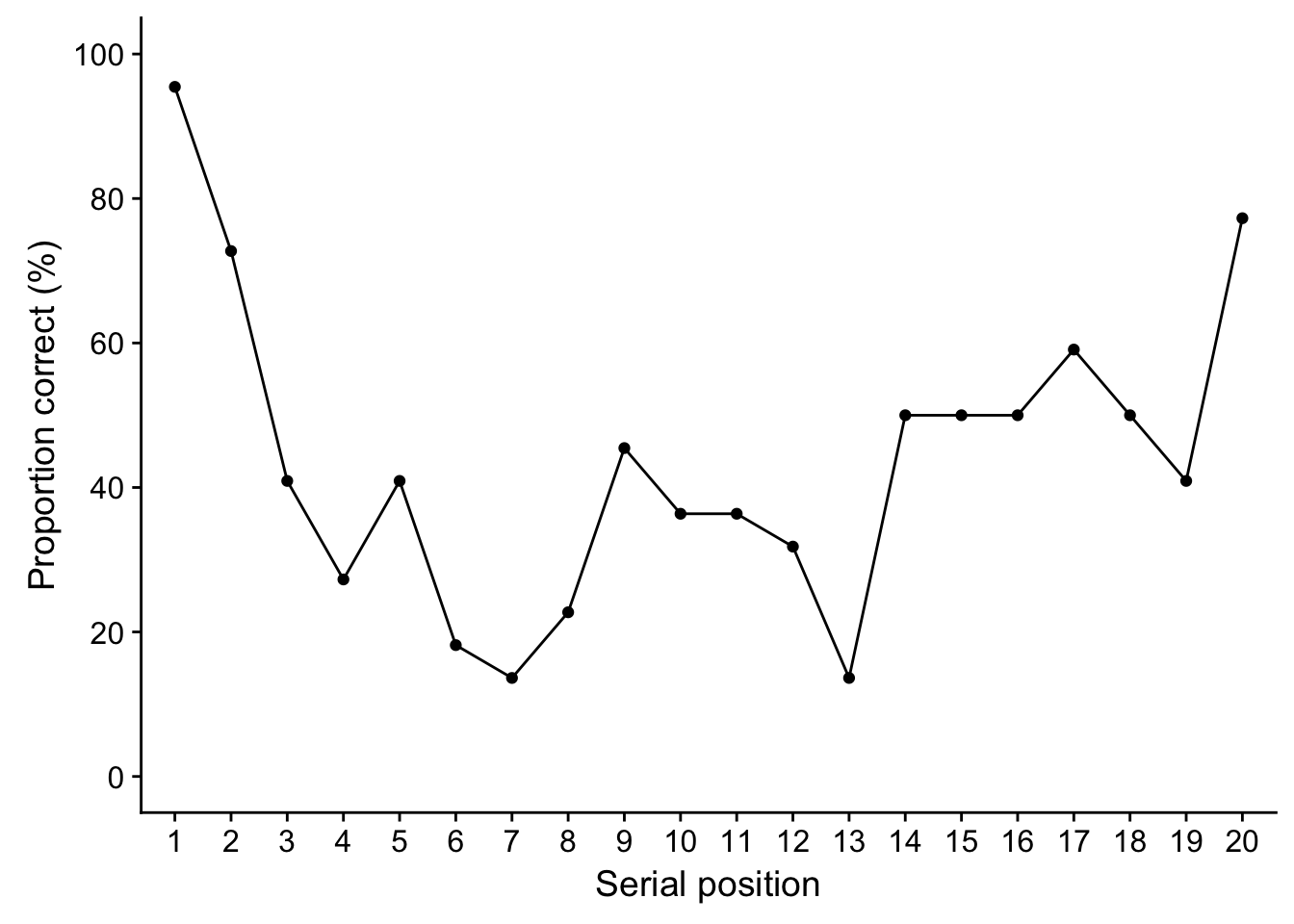I recently taught a class which involved demonstrating the serial position effect. This is the observation that if you present people a list of words, they tend to be better at recalling the beginning and end of the list. This is known as the primacy and recency effect. The first words have been rehearsed and are available in your long-term memory, and the last words are still available in your working memory. I thought the power of R would allow the results to be quickly plotted like a textbook example with a bit of prior preparation.

Before the class, I prepared a table in MS Word (available here along with the plain R script and .csv file) to collect the data which had a list of 20 words. I read the words out in class, and then the students wrote down how many words they could remember. I then passed around a sheet with the words in order and had the students peer mark and write down which words they could remember. During the break I then copied the results down into a .csv file to use with this R script I had prepared. For a class of 22, this took around 15 minutes to enter so it’s best to structure it around a break or while the class are completing another task.

This is how the data looks (after a few packages are loaded). The first column is just a list for the number of students, and then a separate column for each word (note the words aren’t used here, just the serial position). Correctly recalling the word is coded as 1 and an incorrect response is coded as 0 to make the analysis really simple later. To stop it taking up the whole screen, lets take a look at what the first six columns look like.

require(tidyverse)
require(cowplot)

head(serial.dat[, 1:6])
## # A tibble: 6 x 6
##   Participant   SP1   SP2   SP3   SP4   SP5
##         <int> <int> <int> <int> <int> <int>
## 1           1     1     1     0     0     0
## 2           2     1     1     0     0     0
## 3           3     1     0     0     0     1
## 4           4     1     1     0     0     0
## 5           5     1     1     1     0     1
## 6           6     1     1     1     0     1

We can now start preparing the data for plotting. First, we’re going to convert it to long format so we have a column for the participant, the serial position of the word, and whether they remembered the word. We should now have 20 rows of data for each participant.

serial_long <- gather(serial.dat, "serial_position", "Correct", -Participant)

# the ordering can get messed up later on, so it's important to convert the position
# to an ordered factor
serial_long$serial_position <- factor(serial_long$serial_position,
levels = paste("SP", 1:20, sep = ""),
ordered = T)

head(serial_long)
## # A tibble: 6 x 3
##   Participant serial_position Correct
##         <int> <ord>             <int>
## 1           1 SP1                   1
## 2           2 SP1                   1
## 3           3 SP1                   1
## 4           4 SP1                   1
## 5           5 SP1                   1
## 6           6 SP1                   1

The final step for preparing the data for plotting is to calculate the proportion of correct responses for each serial position. As we coded correct recall as a 1, we can simply take the mean of the responses to get the proportion of correct responses. We now have 20 rows, one for each serial position from 1-20, and the proportion of correct responses across the students.

serial_long <- serial_long %>%
group_by(serial_position) %>%
summarise(prop_correct = mean(Correct))

head(serial_long)
## # A tibble: 6 x 2
##   serial_position prop_correct
##   <ord>                  <dbl>
## 1 SP1                    0.955
## 2 SP2                    0.727
## 3 SP3                    0.409
## 4 SP4                    0.273
## 5 SP5                    0.409
## 6 SP6                    0.182

This can finally be plotted to produce something similar to textbook examples of the serial position effect. This class of 22 reproduced the effect pretty well to show the primacy and recency effect.

serial_long %>%
ggplot(aes(x = serial_position, y = prop_correct*100, group = 1)) +
geom_point() +
geom_line() +
ylab("Proportion correct (%)") +
xlab("Serial position") +
scale_y_continuous(limits = c(0, 100),
breaks = seq(0, 100, 20)) +
scale_x_discrete(breaks = paste("SP", 1:20, sep = ""),
labels = as.character(1:20))